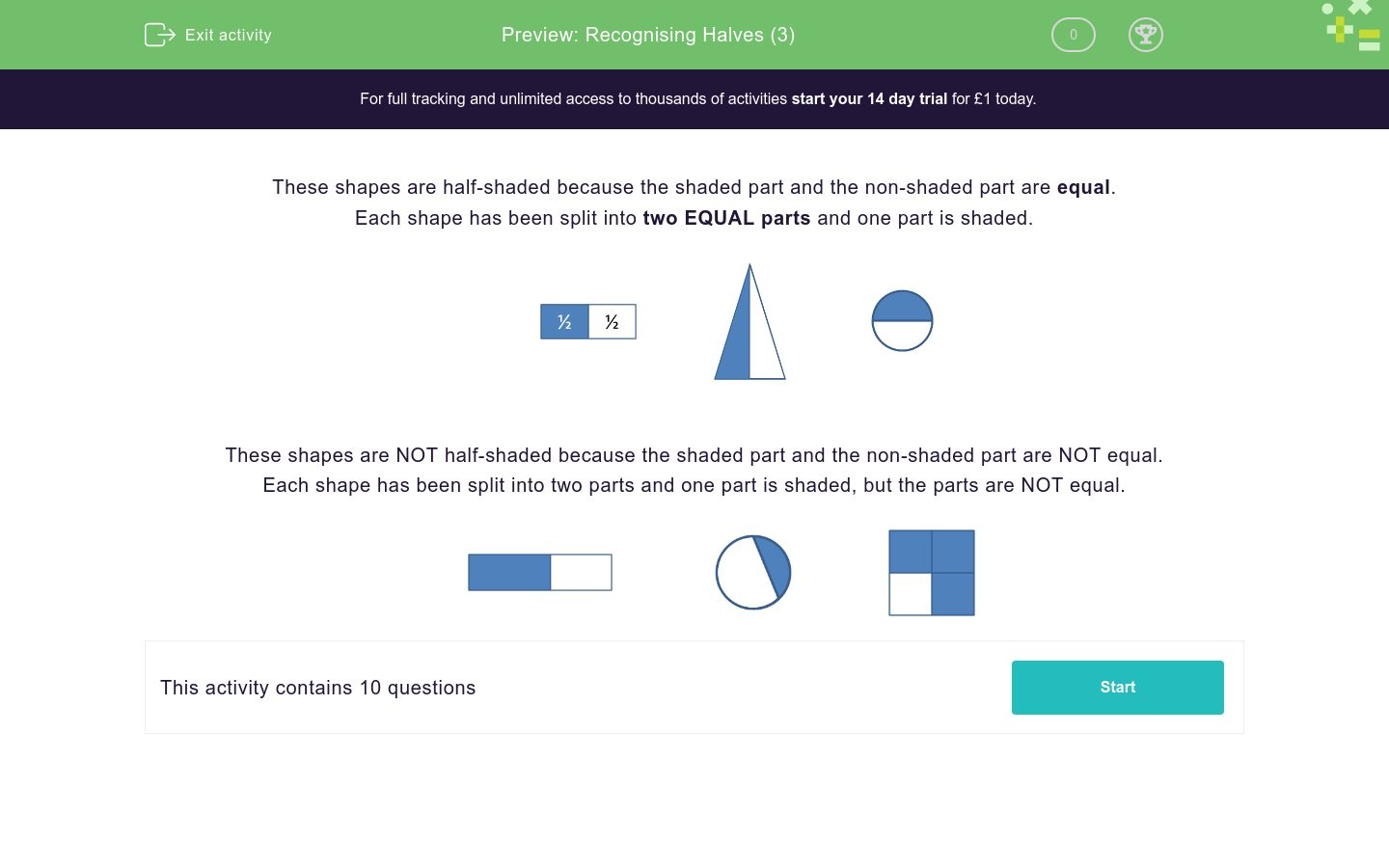# Recognising Halves (3)

In this worksheet, students must state whether a shape is shown half-shaded or not.Key stage:  KS 1

Curriculum topic:   Number: Fractions

Curriculum subtopic:   Recognise Halves and Quarters

Difficulty level:### QUESTION 1 of 10

Each shape has been split into two EQUAL parts and one part is shaded.Each shape has been split into two parts and one part is shaded, but the parts are NOT equal.Select all the shapes which are half-shaded.A B C
A

B

C

Select all the shapes which are half-shaded.A B C
A

B

C

Select all the shapes which are half-shaded.A B C
A

B

C

Select all the shapes which are half-shaded.A B C
A

B

C

Select all the shapes which are half-shaded.A B C
A

B

C

Select all the shapes which are half-shaded.A B C
A

B

C

Select all the shapes which are half-shaded.A B C
A

B

C

Select all the shapes which are half-shaded.A B C
A

B

C

Select all the shapes which are half-shaded.A B C
A

B

C

Select all the shapes which are half-shaded.A B C
A

B

C

• Question 1

Select all the shapes which are half-shaded.A B C
A
B
EDDIE SAYS
A: 2 equal parts and 1 is shaded.
B: 2 equal parts and 1 is shaded.
C: 2 unequal parts.
• Question 2

Select all the shapes which are half-shaded.A B C
B
C
EDDIE SAYS
A: 2 unequal parts.
B: 2 equal parts and 1 is shaded.
C: 2 equal parts and 1 is shaded.
• Question 3

Select all the shapes which are half-shaded.A B C
C
EDDIE SAYS
A: 2 unequal parts.
B: 4 equal parts and 3 are shaded.
C: 2 equal parts and 1 is shaded.
• Question 4

Select all the shapes which are half-shaded.A B C
A
B
C
EDDIE SAYS
A: 2 equal parts and 1 is shaded.
B: 2 equal parts and 1 is shaded.
C: 2 equal parts and 1 is shaded.
• Question 5

Select all the shapes which are half-shaded.A B C
A
C
EDDIE SAYS
A: 2 equal parts and 1 is shaded.
B: 2 unequal parts.
C: 2 equal parts and 1 is shaded.
• Question 6

Select all the shapes which are half-shaded.A B C
B
C
EDDIE SAYS
A: 2 unequal parts.
B: 2 equal parts and 1 is shaded.
C: 2 equal parts and 1 is shaded.
• Question 7

Select all the shapes which are half-shaded.A B C
A
B
C
EDDIE SAYS
A: 2 equal parts and 1 is shaded.
B: 2 equal parts and 1 is shaded.
C: 2 equal parts and 1 is shaded.
• Question 8

Select all the shapes which are half-shaded.A B C
C
EDDIE SAYS
A: 4 equal parts and 1 is shaded.
B: 2 unequal parts.
C: 2 equal parts and 1 is shaded.
• Question 9

Select all the shapes which are half-shaded.A B C
A
B
C
EDDIE SAYS
A: 2 equal parts and 1 is shaded.
B: 2 equal parts and 1 is shaded.
C: 2 equal parts and 1 is shaded.
• Question 10

Select all the shapes which are half-shaded.A B C
C
EDDIE SAYS
A: 2 unequal parts.
B: 2 unequal parts.
C: 2 equal parts and 1 is shaded.
---- OR ----

Sign up for a £1 trial so you can track and measure your child's progress on this activity.

### What is EdPlace?

We're your National Curriculum aligned online education content provider helping each child succeed in English, maths and science from year 1 to GCSE. With an EdPlace account you’ll be able to track and measure progress, helping each child achieve their best. We build confidence and attainment by personalising each child’s learning at a level that suits them.

Get started